# Permutator

(diff) ← Older revision | Latest revision (diff) | Newer revision → (diff)
An eigen valueof a stochastic kernel that it is different from one and such that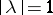. A non-negative continuous function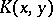given on a compact setin a finite-dimensional space is called a stochastic kernel if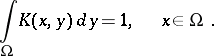The eigen values of such a kernel satisfy the condition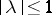. In operator theory, the name permutator is also given to an operator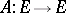if the range of its values,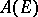, is finite dimensional and if there exists a basisinsuch that,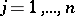.